On-line GuidesAll GuideseBook StoreiOS / AndroidLinux for BeginnersOffice ProductivityLinux InstallationLinux SecurityLinux UtilitiesLinux VirtualizationLinux KernelSystem/Network AdminProgrammingScripting LanguagesDevelopment ToolsWeb DevelopmentGUI Toolkits/DesktopDatabasesMail SystemsopenSolarisEclipse DocumentationTechotopia.comVirtuatopia.comHow To GuidesVirtualizationGeneral System AdminLinux SecurityLinux FilesystemsWeb ServersGraphics & DesktopPC HardwareWindowsProblem SolutionsPrivacy Policy1. Evaluate These Expressions. The following expressions are somewhat more complex, and use functions from the math module.

`math.sqrt( 40.0/3.0 - math.sqrt(12.0) )`

`6.0/5.0*( (math.sqrt(5)+1) / 2 )**2`

`math.log( 2198 ) / math.sqrt( 6 )`

2. Run demorandom.py. Run the `demorandom.py` script several times and save the results. Then add the following statement to the script and run it again several times. What happens when we set an explicit seed?

```#!/usr/bin/env python
import random
random.seed(1)

...everything else the same

```

Try the following variation, and see what it does.

```#!/usr/bin/env python
import random, time
random.seed(time.clock())

...everything else the same

```
3. Wind Chill. Wind chill is used by meteorologists to describe the effect of cold and wind combined.

Given the wind speed in miles per hour, v , and the temperature in °F, t , the Wind Chill, w , is given by the formula below.

Equation 5.1. Wind Chill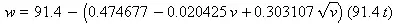Wind speeds are for 0 to 40 mph, above 40, the difference in wind speed doesn't have much practical impact on how cold you feel.

Write a print statement to compute the wind chill felt when it is -2 °F and the wind is blowing 15 miles per hour.

4. How Much Does The Atmosphere Weigh? From Slicing Pizzas, Racing Turtles, and Further Adventures in Applied Mathematics, [Banks02].

Air Pressure (at sea level) P0 = 1.01325×105 newtons/m2. A newton is 1 kg·m/sec2 or the force acting on a kg of mass.

Gravity acceleration (at sea level) g = 9.82m/sec2. This converts force from newtons to kg of mass.

Equation 5.2. Mass of air per square meter (in Kg)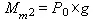Given the mass of air per square meter, we need to know how many square meters of surface to apply this mass to.

Radius of Earth R = 6.37×106m.

Equation 5.3. Area of a Sphere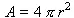Equation 5.4. Mass of atmosphere (in Kg)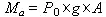Check: somewhere around 1018kg.

5. How much does the atmosphere weigh? Part 2. From Slicing Pizzas, Racing Turtles, and Further Adventures in Applied Mathematics, [Banks02].

The exercise How Much Does The Atmosphere Weigh? assumes the earth to be an entirely flat sphere. The averge height of the land is actually 840m. We can use the ideal gas law to compute the pressure at this elevation and refine the number a little further.

Equation 5.5. Pressure at a given elevation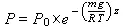molecular weight of air m = 28.96×10-3 kg/mol.

gas constant R = 8.314 joule/°Kmol.

gravity g = 9.82 m/sec2.

temperature (°K) T = 273°+°C (assume 15°C for temperature).

elevation z = 840 m.

This pressure can be used for the air over land, and the pressure computed in How Much Does The Atmosphere Weigh? can be used for the air over the oceans. How much land has this reduced pressure? Reference material gives the ocean area ( Ao = 3.61×1014 m2) and the land area ( Al = 1.49×1014 m2).

Equation 5.6. Weight of Atmosphere, adjusted for land elevation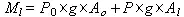Published under the terms of the Open Publication License Design by Interspire